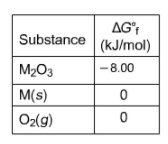# Problem: Consider the decomposition of a metal oxide to its elements, where M represents a generic metal.M2O3(s) ⇌ 2 M(s) + 3/2 O2(g)What is the standard change in Gibbs energy for the reaction, as written, in the forward direction? What is the equilibrium constant of this reaction, as written, in the forward direction at 298 K?What is the equilibrium pressure of O2(g) over M(s) at 298 K?

###### FREE Expert Solution

Part 1) We're being asked to calculate the standard change in Gibbs energy for the reaction.

M2O3(s) ⇌ 2 M(s) + 3/2 O2(g)

We can use the following equation to solve for ΔG˚rxn:

The values for ΔG˚f:

ΔG˚f, M2O3(s) = –8.00 kJ/mol

ΔG˚f, M(s) = 0 kJ/mol

ΔG˚f, O2(g) = 0 kJ/mol

Note that we need to multiply each ΔG˚f by the stoichiometric coefficient since ΔG˚f is in kJ/mol.

90% (328 ratings)###### Problem Details

Consider the decomposition of a metal oxide to its elements, where M represents a generic metal.

M2O3(s) ⇌ 2 M(s) + 3/2 O2(g)What is the standard change in Gibbs energy for the reaction, as written, in the forward direction?

What is the equilibrium constant of this reaction, as written, in the forward direction at 298 K?

What is the equilibrium pressure of O2(g) over M(s) at 298 K?

Frequently Asked Questions

What scientific concept do you need to know in order to solve this problem?

Our tutors have indicated that to solve this problem you will need to apply the Gibbs Free Energy concept. You can view video lessons to learn Gibbs Free Energy. Or if you need more Gibbs Free Energy practice, you can also practice Gibbs Free Energy practice problems.